# Lebesgue spectrum

(diff) ← Older revision | Latest revision (diff) | Newer revision → (diff)

A term in spectral theory. Letbe a self-adjoint anda unitary operator acting in a Hilbert space. The operator, respectively, has a simple Lebesgue spectrum if it is unitarily equivalent to the operator of multiplication byin a space of complex-valued functions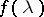that are defined on the real axis, respectively on the circleand for which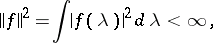where the integration is carried out with respect to the ordinary Lebesgue measure on, respectively on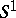; hence the name Lebesgue spectrum (see Unitarily-equivalent operators). Forthis definition is equivalent to the following: Inthere is an orthonormal basis,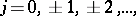such that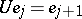. Also, an operator has a Lebesgue spectrum ifcan be decomposed into an orthogonal direct sum of invariant subspaces in each of which the operator has a simple Lebesgue spectrum. Although for a given operator there can be many such decompositions, the number of "summands" in each of them is the same (it may be an infinite cardinal number). This number is called the multiplicity of the Lebesgue spectrum. Finally, similar concepts can be introduced for one-parameter groups of unitary operators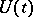, continuous in the weak (or strong, which is the same in the given case) operator topology. By Stone's theorem,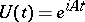, whereis a self-adjoint operator (cf. Semi-group of operators; Generating operator of a semi-group). Ifhas a Lebesgue spectrum of a certain multiplicity, one says thathas the same properties. For example, the grouphas a simple Lebesgue spectrum if it is unitarily equivalent to the group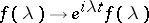in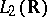, and this group, in turn, is equivalent to the group of shiftsin the same space.